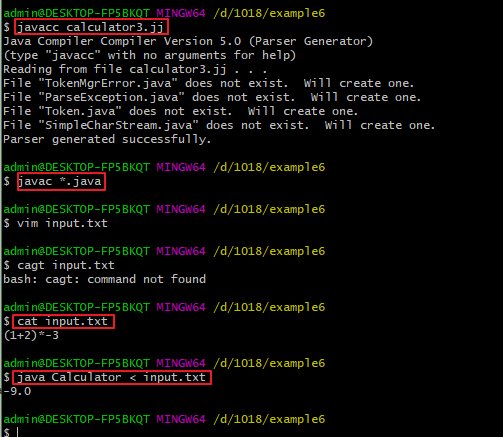# 例6：计算器--添加括号、一元运算符和历史记录

## 1.calculator3.jj

我们只需要再添加一些特色，就可以得到一个可用的四则运算计算器。在这一版的修改中 ，我们将使得程序可以接收括号、负值，并且还可以通过$符号来引用上一次计算的结果。 对词法描述文件的修改如下所示，我们只添加下面3行： TOKEN : { < OPEN_PAR : "(" > } TOKEN : { < CLOSE_PAR : ")" > } TOKEN : { < PREVIOUS : "$" > }


我们没有必要专门为负号创建一个token，因为我们已经定义了MINUS这个token了。
对于语法描述部分的修改，则都是体现在了Primary当中，在Primary当中有4中可能的值：一个数值（跟之前的例子一样）、一个$符号、带有括号的表达式、一个负号然后跟着前3个的任一种。BNF符号表达式如下： Primary --> NUMBER | PREVIOUS | OPEN_PAR Expression CLOSE_PAR | MINUS Primary  这个BNF生产式中有两个递归。最后一种选择是直接递归，倒数第二个选择是间接递归，因为Expression最终还是依赖于Primary。在BNF生产式中使用递归是没有任何问题的，当然也有一些限制，我们将在后面谈论到。 考虑下面的表达式： - - 22  那么Primary就是下图中的方块部分：在语法分析器执行到上面的输入时，对于每一个方块，都会调用一次Primary。相似的，对于下面的输入： 12 * ( 42 + 19 )  我们把Primary框出来，可得到如下所示：相互嵌套的框框，其实就表示了相互递归调用的Primary方法。 下面是JavaCC中Primary的生产式： double Primary() throws NumberFormatException : { Token t ; double d ; } { t=<NUMBER> { return Double.parseDouble( t.image ) ; } | <PREVIOUS> { return previousValue ; } | <OPEN_PAR> d=Expression() <CLOSE_PAR> { return d ; } | <MINUS> d=Primary() { return -d ; } }  ## 2. 测试 根据上面的修改，得到完整的calculator3.jj文件如下： /* calculator0.jj An interactive calculator. */ options { STATIC = false ; } PARSER_BEGIN(Calculator) import java.io.PrintStream ; class Calculator { public static void main( String[] args ) throws ParseException, TokenMgrError, NumberFormatException { Calculator parser = new Calculator( System.in ) ; parser.Start( System.out ) ; } double previousValue = 0.0 ; } PARSER_END(Calculator) SKIP : { " " } TOKEN : { < EOL : "\n" | "\r" | "\r\n" > } TOKEN : { < PLUS : "+" > } TOKEN : { < MINUS : "-" > } TOKEN : { < TIMES : "*" > } TOKEN : { < DIVIDE : "/" > } TOKEN : { < OPEN_PAR : "(" > } TOKEN : { < CLOSE_PAR : ")" > } TOKEN : { < PREVIOUS : "$" > }
TOKEN : { < NUMBER : <DIGITS>
| <DIGITS> "." <DIGITS>
| <DIGITS> "."
| "."<DIGITS> >
}
TOKEN : { < #DIGITS : (["0"-"9"])+ > }

void Start(PrintStream printStream) throws NumberFormatException :
{}
{
(
previousValue = Expression()
<EOL> { printStream.println( previousValue ) ; }
)*
<EOF>
}

double Expression() throws NumberFormatException :
{
double i ;
double value ;
}
{
value = Term()
(
<PLUS>
i = Term()
{ value += i ; }
| <MINUS>
i = Term()
{ value -= i ; }
)*
{ return value ; }
}

double Term() throws NumberFormatException :
{
double i ;
double value ;
}
{
value = Primary()
(
<TIMES>
i = Primary()
{ value *= i ; }
| <DIVIDE>
i = Primary()
{ value /= i ; }
)*
{ return value ; }
}

double Primary() throws NumberFormatException :
{
Token t ;
double d ;
}
{
t=<NUMBER>
{ return Double.parseDouble( t.image ) ; }
|   <PREVIOUS>
{ return previousValue ; }
|   <OPEN_PAR> d=Expression() <CLOSE_PAR>
{ return d ; }
|   <MINUS> d=Primary()
{ return -d ; }
}计算器示例到此结束。官方文档中其实还有一个“文本处理”的例子，时间有限就不翻译了。可以直接去阅读英文原版，其实还是比较容易懂的。上面的示例通过由浅入深的引导，让我们大致知道JavaCC能干什么以及是怎么工作的。如果想要使用JavaCC做更多的事情，建议还是把最后一个例子的英文原版看完看懂，并多加练习。

posted @ 2019-10-24 17:43  象牙酥  阅读(1191)  评论(0编辑  收藏